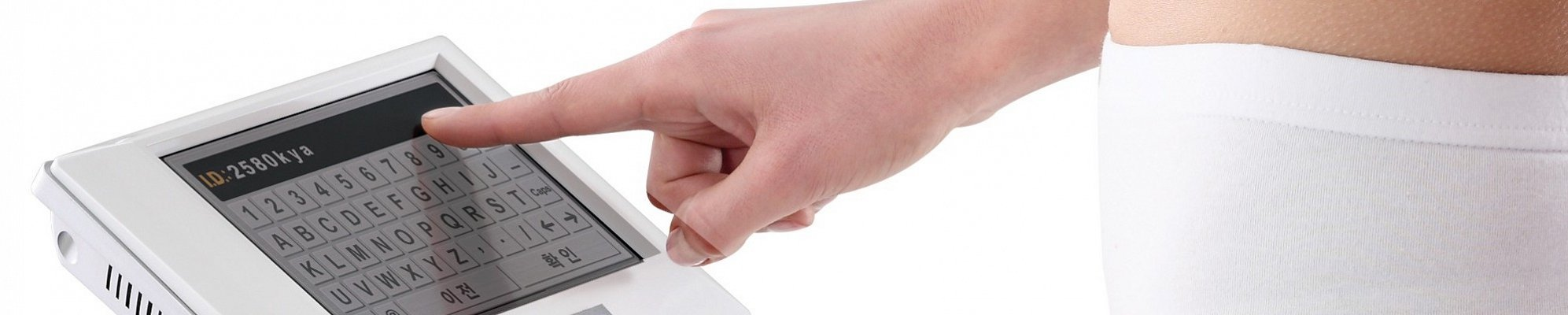# How does the scan work?

Unless you have a degree in physics, - it’s complicated.

If you’re interested in the science of body composition analysis, the following information will go some way to explaining how it all works - or alternatively watch this video.

The Bodystat 1500mdd measures composition using Bioelectrical Impedance Analysis (BIA).

Bioelectrical Impedance Analysis (BIA) measures the impedance (or opposition) to the flow of an electric current through the body fluids contained mainly in the lean and fat tissue.

Impedance is LOW in lean tissue, where intracellular and extracellular fluid and electrolytes are primarily contained, but HIGH in fat tissue.

In plain English, the electric current emitted from the scanner (which you won’t feel during the test) easily passes through water (of which there is plenty of in the muscles) and not very easily through fat. From these readings, it’s possible to get an accurate idea of what your hydration, body fat and lean tissue levels are.

Impedance is proportional to body water volume (TBW).

## Impedance, lean tissue and body water

In practice, a small constant current, typically 400 uA at a fixed or multi frequencies are passed between electrodes attached to your hand and foot and the voltage drop between electrodes provides a measure of impedance.

Prediction equations, previously generated by correlating impedance measured against an independent estimate of TBW, may be used subsequently to convert a measured impedance to a corresponding estimate of TBW.

Lean body mass is then calculated from this estimate using an assumed hydration fraction for lean tissue.

(NOTE: The Bodystat we use is unique in using it’s own regression equation for this calculation and not the assumed 73.2% used by other manufacturers scanners. Fat mass is calculated as the difference between body weight and lean body mass.

## Measuring "Intra" and "extra" cellular hydration

The impedance of a biological tissue comprises two components, the resistance and the reactance.

The conductive characteristics of body fluids provide the resistive component, whereas the cell membranes, acting as imperfect capacitors, contribute a frequency-dependent reactive component.

By measuring the impedance at 50 kHz and 200 kHz and by applying predictive equations, it is possible to estimate both extra-cellular water (ECW) and TBW respectively and by deduction, intra-cellular water (ICW). ECW can be related to extra-cellular mass (ECM) and ICW to body cell mass (BCM).

Hopefully that goes some way to explaining the science behind the body scans we offer, but if you are more into “visual” explanations, take a look at this video.